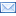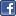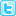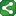Wednesday, July 22, 2009

Renaming All Variables in a SAS Data Set Using the SASHELP VIEWS

*Create a temporary dataset... DSN;
data dsn;
a=1;
b=2;
c=3;
d=4;
e=5;
f=6;
run;

%macro test(lib,dsn);

*/1)*/ data _null_;
set sashelp.vtable(where=(libname="&LIB" and memname="&DSN"));
call symput('nvars',nvar);
run;

*/2)*/ data dsn;
set sashelp.vcolumn(where=(libname="&LIB" and memname="&DSN"));
call symput(cats("var",_n_),name);
run;

*/3)*/ proc datasets library=&LIB;
modify &DSN;
rename
%do i = 1 %to &nvars;
&&var&i=Rename_&&var&i.
%end;
;
quit;
run;
%mend;

%test(WORK,DSN);

After submitting the above program... the output looks like this....

Output:
Rename_a Rename_b Rename_c Rename_d Rename_e Rename_f
1 2 3 4 5 6

Here is a way I know of.. to rename all the variables in the dataset;

It can be done using the SASHELP views as follows:

1) The 1st step of the program determines the total number of variables inside the dataset with the help of SASHELP.Vtable, Data _null_ step used to do the same …. A macro variable NVAR (Number of Variables) will be created after this step which can be accessed in the following steps.

2) What the 2nd step does is….assigns a unique IDNUM to all the variables of the dataset with the help of SASHELP.Vcolumn.

3) What the 3rd step does is … it uses Proc Datasets with the MODIFY statement to rename all the variables in the dataset. The DO LOOP is been used to resolve the 6 macro variables in a macro of a temporary dataset.ShareThis